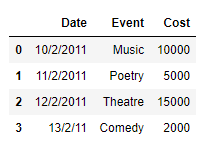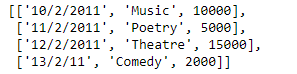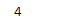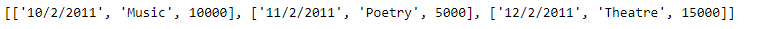# Create a list from rows in Pandas DataFrame | Set 2

In an earlier post, we had discussed some approaches to extract the rows of the dataframe as a Python’s list. In this post, we will see some more methods to achieve that goal.

Solution #1: In order to access the data of each row of the Pandas dataframe, we can use `DataFrame.iloc` attribute and then we can append the data of each row to the end of the list.

 `# importing pandas as pd ` `import` `pandas as pd ` ` `  `# Create the dataframe ` `df ``=` `pd.DataFrame({``'Date'``:[``'10/2/2011'``, ``'11/2/2011'``, ``'12/2/2011'``, ``'13/2/11'``], ` `                    ``'Event'``:[``'Music'``, ``'Poetry'``, ``'Theatre'``, ``'Comedy'``], ` `                    ``'Cost'``:[``10000``, ``5000``, ``15000``, ``2000``]}) ` ` `  `# Print the dataframe ` `print``(df) `

Output :Now we will use the `DataFrame.iloc` attribute to access the values of each row in the dataframe and then we will construct a list out of it.

 `# Create an empty list ` `Row_list ``=``[] ` ` `  `# Iterate over each row ` `for` `i ``in` `range``((df.shape[``0``])): ` ` `  `    ``# Using iloc to access the values of  ` `    ``# the current row denoted by "i" ` `    ``Row_list.append(``list``(df.iloc[i, :])) ` ` `  `# Print the list ` `print``(Row_list) `

Output :As we can see in the output, we have successfully extracted each row of the given dataframe into a list. Just like any other Python’s list we can perform any list operation on the extracted list.

 `# Find the length of the newly  ` `# created list ` `print``(``len``(Row_list)) ` ` `  `# Print the first 3 elements ` `print``(Row_list[:``3``]) `

Output :Solution #2: In order to access the data of each row of the Pandas dataframe we can use `DataFrame.iat` attribute and then we can append the data of each row to the end of the list.

 `# importing pandas as pd ` `import` `pandas as pd ` ` `  `# Create the dataframe ` `df ``=` `pd.DataFrame({``'Date'``:[``'10/2/2011'``, ``'11/2/2011'``, ``'12/2/2011'``, ``'13/2/11'``], ` `                    ``'Event'``:[``'Music'``, ``'Poetry'``, ``'Theatre'``, ``'Comedy'``], ` `                    ``'Cost'``:[``10000``, ``5000``, ``15000``, ``2000``]}) ` ` `  `# Create an empty list ` `Row_list ``=``[] ` ` `  `# Iterate over each row ` `for` `i ``in` `range``((df.shape[``0``])): ` `    ``# Create a list to store the data ` `    ``# of the current row ` `    ``cur_row ``=``[] ` `     `  `    ``# iterate over all the columns ` `    ``for` `j ``in` `range``(df.shape[``1``]): ` `         `  `        ``# append the data of each ` `        ``# column to the list ` `        ``cur_row.append(df.iat[i, j]) ` `         `  `    ``# append the current row to the list ` `    ``Row_list.append(cur_row) ` ` `  `# Print the list ` `print``(Row_list) `

Output :`# Find the length of the newly  ` `# created list ` `print``(``len``(Row_list)) ` ` `  `# Print the first 3 elements ` `print``(Row_list[:``3``]) `

Output :My Personal Notes arrow_drop_upCheck out this Author's contributed articles.

If you like GeeksforGeeks and would like to contribute, you can also write an article using contribute.geeksforgeeks.org or mail your article to contribute@geeksforgeeks.org. See your article appearing on the GeeksforGeeks main page and help other Geeks.

Please Improve this article if you find anything incorrect by clicking on the "Improve Article" button below.

Article Tags :

Be the First to upvote.

Please write to us at contribute@geeksforgeeks.org to report any issue with the above content.